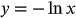1
35
views
61c
Problem

Problem 61c

Chapter 1: Functions and ModelsTextbook ExpertVerified Tutor
18 Oct 2021

Given information

The given equation is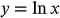.

Reflect the graph of the given equation about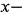axis.

Step-by-step explanation

Step 1.

Transformation rule:

The graph of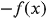is the reflection of the graph of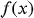aboutaxis.

Draw the graph ofand then reflect the graph aboutaxis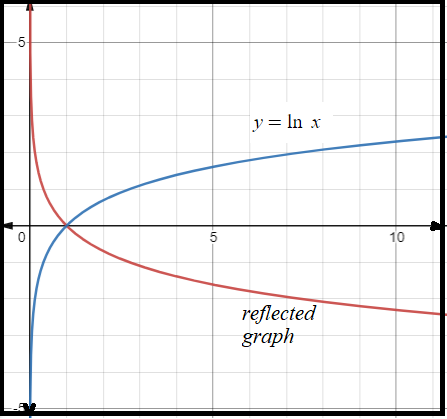From the above graph the equation of the reflected graph is